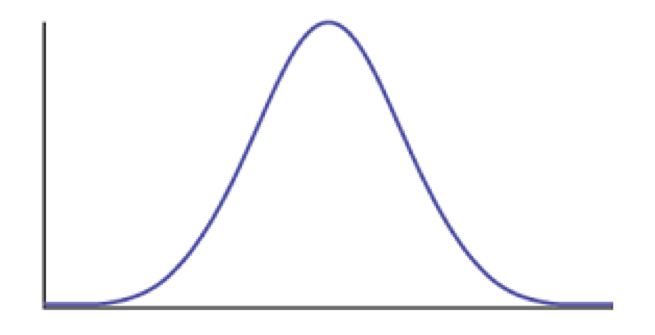# 6.4: Non-Brownian evolution under stabilizing selection

•• Contributed by Luke J. Harmon
• Professor (Biological Sciences) at University of Idaho

We can also consider the case where a trait evolves under the influence of stabilizing selection. Assume that a trait has some optimal value, and that when the population mean differs from the optimum the population will experience selection towards the optimum (Figure 6.3). As I will show below, when traits evolve under stabilizing selection with a constant optimum, the pattern of traits through time can be described using an Ornstein-Uhlenbeck (OU) model. It is worth mentioning, though, that this is only one (of many!) models that follow an OU process over long time scales. In other words, even though this model can be described by OU, we cannot make inferences the other direction and claim that OU means that our population is under constant stabilizing selection. In fact, we will see later that we can almost always rule this simple version of the OU model out over long time scales by looking at the actual parameter values of the model compared to what we know about species’ population sizes and trait heritabilities.Figure 6.3. Plot of species trait (x axis) versus fitness (y axis) showing a hypothetical landscape that would produce stabilizing selection. Image by the author, can be reused under a CC-BY-4.0 license.

We can follow the modeling approach from chapter 3 to derive the expected distribution of species’ traits on a tree under stabilizing selection. The derivation is a bit long and complicated, so I have moved it to an appendix of this chapter. For now, all you need to know is that we can write down the likelihood of an OU model on a phylogenetic tree (see equation 6.58-6.60, below).

We can fit an OU model to data in a similar way to how we fit BM models in the previous chapters. For any given parameters ($$\bar{z}_0$$, σ2, α, and θ) and a phylogenetic tree with branch lengths, one can calculate an expected vector of species means and a species variance-covariance matrix. One then uses the likelihood equation for a multivariate normal distribution to calculate the likelihood of this model. This likelihood can then be used for parameter estimation in either a ML or a Bayesian framework.

We can illustrate how this works by fitting an OU model to the mammal body size data that we have been discussing. Using ML, we obtain parameter estimates $$\hat{\bar{z}}_0 = 4.60$$, $$\hat{\sigma}^2 = 0.10$$, $$\hat{\alpha} = 0.0082$$, and $$\hat{\theta} = 4.60$$. This model has a lnL of -77.6, a little higher than BM, but an AICc score of 161.2, worse than BM. We still prefer Brownian motion for these data. Over many datasets, though, OU models fit better than Brownian motion (see Harmon et al. 2010; Pennell and Harmon 2013).